# Kähler-Einstein manifold

A complex manifold carrying a Kähler–Einstein metric. By the uniqueness property of Kähler–Einstein metrics (see [a2], [a4]), the concept of a Kähler–Einstein manifold provides a very natural tool in studying the moduli space of compact complex manifolds.

## Examples.

1) Calabi–Yau manifolds. Any compact connected Kähler manifold of complex dimensionwith holonomy in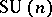is called a Calabi–Yau manifold. A Fermat quintic in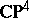with a natural Ricci-flat Kähler metric is a typical example of a Calabi–Yau threefold. Interesting subjects, such as mirror symmetry, have been studied for Calabi–Yau threefolds.

2) More generally, Ricci-flat Kähler manifolds are Kähler–Einstein manifolds (cf. also Ricci curvature). For instance, hyper-Kähler manifolds, characterized as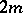-dimensional (possibly non-compact) Kähler manifolds with holonomy in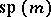, are Ricci-flat Kähler manifolds (see [a1], [a3]). An ALE gravitational instanton, obtained typically as a minimal resolution of an isolated quotient singularity in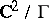, has the structure of a hyper-Kähler manifold. A K3-surface (cf. Surface, K3) is a compact hyper-Kähler manifold.

3) Kähler C-spaces. A compact simply connected homogeneous Kähler manifold, called a Kähler C-space, carries a Kähler–Einstein metric with positive scalar curvature and has the structure of a Kähler–Einstein manifold.

4) A twistor space of a quaternionic Kähler manifold with positive scalar curvature has the natural structure of a Kähler–Einstein manifold with positive scalar curvature (see [a3]).

5) Among the almost-homogeneous Kähler manifolds (cf. [a1]), the hypersurfaces in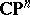and the del Pezzo surfaces (cf. [a5], [a6] or Cubic hypersurface), there are numerous examples of Kähler–Einstein manifolds with positive scalar curvature.

6) Any complex manifold covered by a bounded homogeneous domain inendowed with a Bergman metric (cf. also Hyperbolic metric) is a Kähler–Einstein manifold with negative scalar curvature. More generally, a compact complex manifoldwith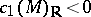naturally has the structure of a Kähler–Einstein manifold with negative scalar curvature.

## Generalization.

A compact complex surface with quotient singularities obtained from a minimal algebraic surface of general type by blowing down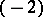-curves has the structure of a Kähler–Einstein orbifold, which is a slight generalization of the notion of a Kähler–Einstein manifold.

General references for Kähler–Einstein manifolds are [a1], [a2] and [a4].